Interacting with a user is one of the great features of programming. If you can't take the inputs from a user, then you cannot take actions based on what the user needs. That's where Python Input Function comes in picture. Moreover, Python has an inbuilt function called input() to cater to user inputs. Let's see the list of the tutorial.

• What is the Python Input function?
• Python Input function argument
• Python Input function return type
• Type Casting in Python

## What is the Python Input function?

We have already seen the print function in Python, which sends data to the console. Python Input function does the reverse of it. In other words, it takes user data from the console so that the program can take action based on that input.

The input function indicates the user to enter the data on the console. Moreover, the program executes further only when the user has entered the data. This data can then be stored in a variable and can use it in the program wherever we need it.

Note - Input function always takes the input data as String. So if you are asking the user to enter age, and he enters age as 25, this will be considered a string. Additionally, you need to convert it to int before you take any action on it.

Write the following in IDE and run it.

``````# taking input from the user
name = input()
print(name)
``````If you run the above program, you will see a blinking cursor in the output console, as shown in the below snap. It is waiting for the data. Moreover, the program won't execute the further steps until we give the input.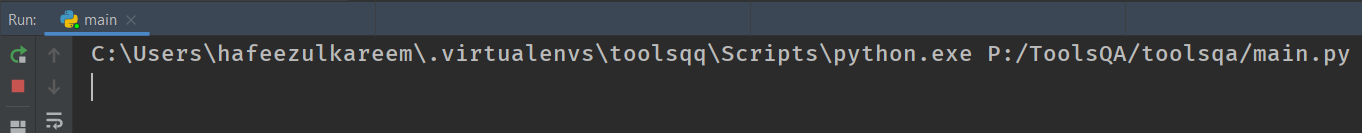Now, enter anything in the console.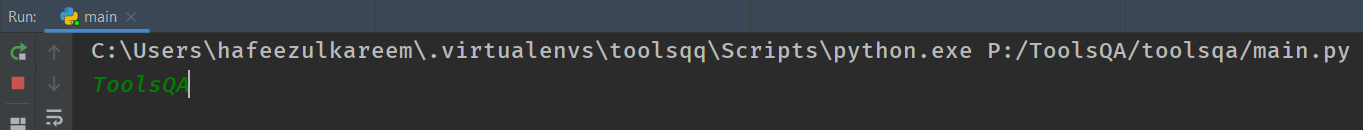After entering any text hit Enter. Consequently, you will get what you entered as output.The storage of the data that we have given is in the variable name. And we have printed the variable in the last line of the code. That's the output we have seen in the above image and your IDE.

### How to pass an argument to the Python Input function?

How does a user know he has to enter a number or name or any other data? To tell what type of data user has to enter, we can pass a message to the input() function that displays to the user.

For example, your program needs the name of the user to print a welcome message. You can write the input statement as input("Enter your name:- "). It displays the message to the user. Subsequently, try the following code in IDE.

``````# getting name of the user
user_name = input("Enter your name:- ")
print("Hi,", user_name, "\b!")
``````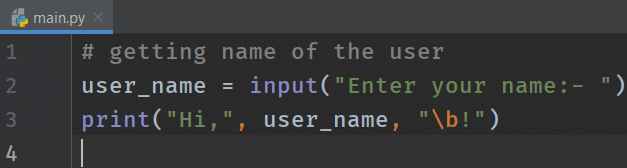We have given a message to the user that Enter your name:-. User will read the message and enter the data accordingly.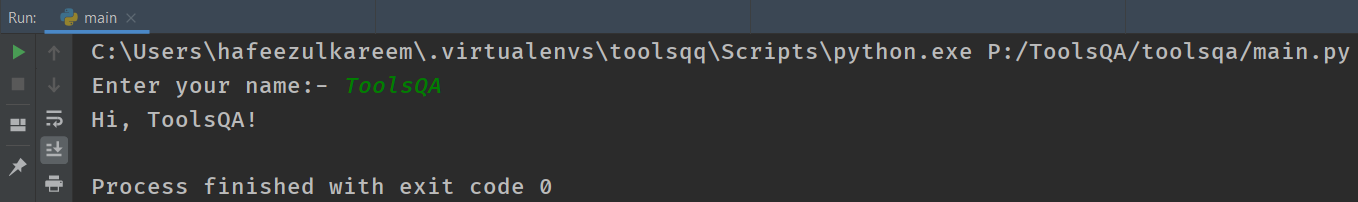You can see that we have stored the user input in a variable user_name, and while printing it, we have added "Hi" to it.

What happens when you pass multiple arguments to the input() function? Will it show everything like print() function?

Let's see what happens with the following code.

``````# input() -> multiple arguments
num = input("Enter a number", "greater than 0 and less than 100")
print(num)
``````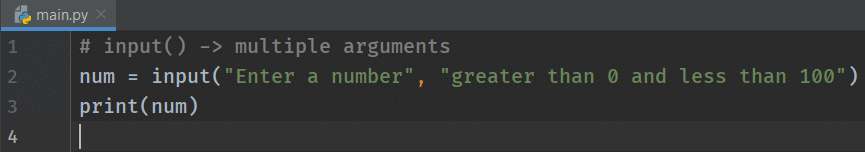We got an error. Why is it? The input() function takes at most one argument, but we passed two arguments to the input function. As a result, we got an error. Remember python user input() function takes only one argument. In other words, if we don't pass anything, then the input function won't show any message to the user, as seen in the first section of the tutorial.

### What is the Return Type of Python Input function?

We have mentioned that the python user input takes the information as a string type. Let's check by an experiment.

``````# getting input from the user
number = input("Enter a number:- ")
result = number + 2
print(result)
``````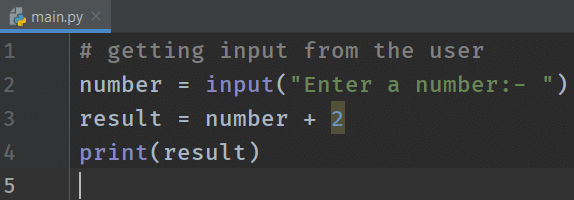What do you expect the output will be? Run the code and enter a number. See what you get.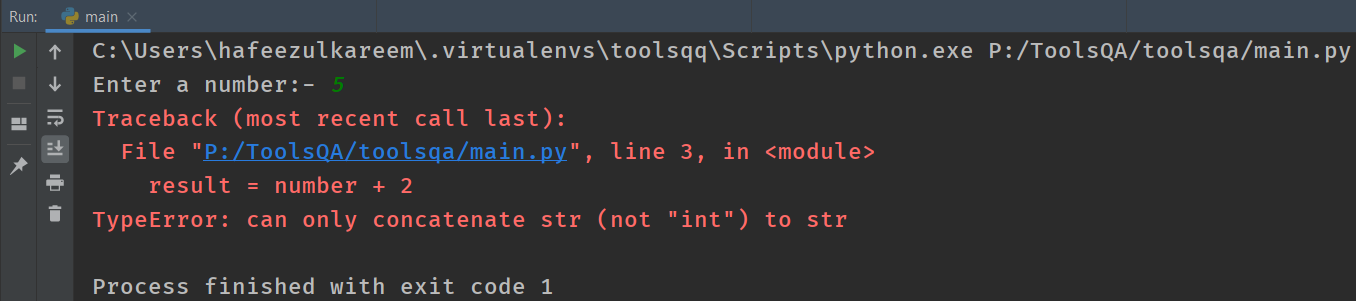We got the error. Why? The data that we got from the console is a string. And we try to add a string to a number. So, we got the error.

What if we want to take a number as input? Is it possible? Let's jump into the next section to find the answers.

## Type Casting

Converting one type of data to other kinds of data is known as typecasting. We are already familiar with such kind of conversions.

What if we want to take an integer, or float as input. Is it possible in Python? The answer is NO. But, we can use the typecasting (type conversion) using built-in data type functions.

Let's try to convert the python string to python integer. You still remember the int function, don't you?

``````# getting input from the user
# and converting it to an integer
# int() function
number = int(input("Enter a number:- "))
result = number + 2
print(result)
``````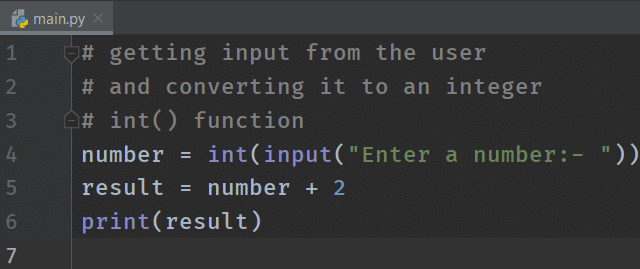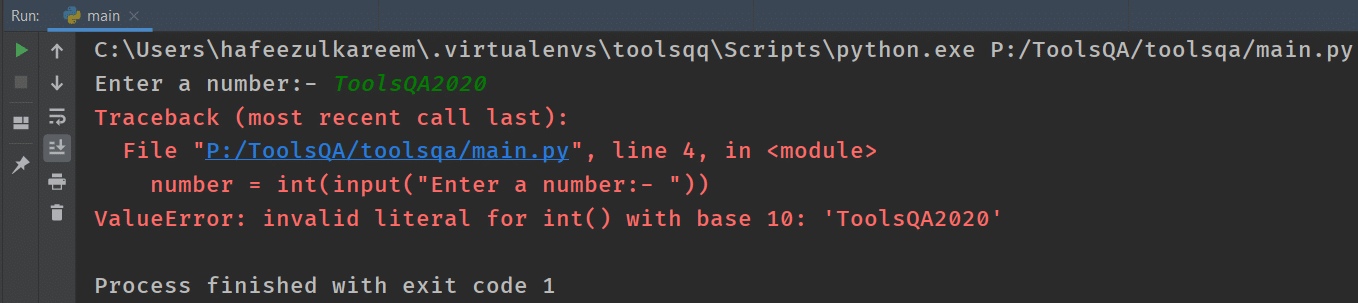We have converted the input data to an integer at the time of taking the input itself. Another option was first to consider the number as a string, store it in a variable, and then convert it to int. While this will work perfectly fine, it will increase the lines of code and memory usage. It's always best to write efficient code!!

We will get an error if we try to convert a string that contains alphabets or other special characters. Run the above code and give the 2020ToolsQA as input.We are trying to convert a string that is not a number. So, Python throws an error.

Similar to the conversion of strings to integers, we can convert them to floats as well. You can use int or float functions for the conversion based on your needs.

That's it for this tutorial.

## Key Takeaways

• input() function takes the data from the console in the form of string.
• Moreover, we can pass a message to the input() function that will appear to the user before giving the data input. E.g. input("Enter a number:- ")
• If we pass multiple arguments to the input() function, then we will get the error.
• input() function returns the data in the string (str) format. Moreover, we can use the typecasting for type conversion. E.g. number = int(input("Enter a number:- "))
• Additionally, converting one type of data into other types of data is known as typecasting.
• We can use the functions int, str, and float for the typecasting.

## Practice Yourself

#Problem 1

``````# input:- ToolsQA
print(input())
``````

#Problem 2

``````# input:- ToolsQA
print("Welcome to " + input() + "!")
``````

#Problem 3

``````# Follow the instructions to write a program
# 1. Take a number as input from the user
# 2. Multiply it with 10 and divide the result by 10
# 3. Print the above the result
``````

Problem 4

``````# Follow the instructions to write a program
# 1. Take the user name as input and store it in a variable
# 2. Take the age of the user as a number and store it in a variable
# 3. Print the a line of string as follows
# Output: Your name is <user-name> and age <user-age>
``````

#Problem 5

``````# Follow the instructions to write a program
# 1. Take two numbers from the user as input
# 2. Add those two numbers
# 3. Print the result
``````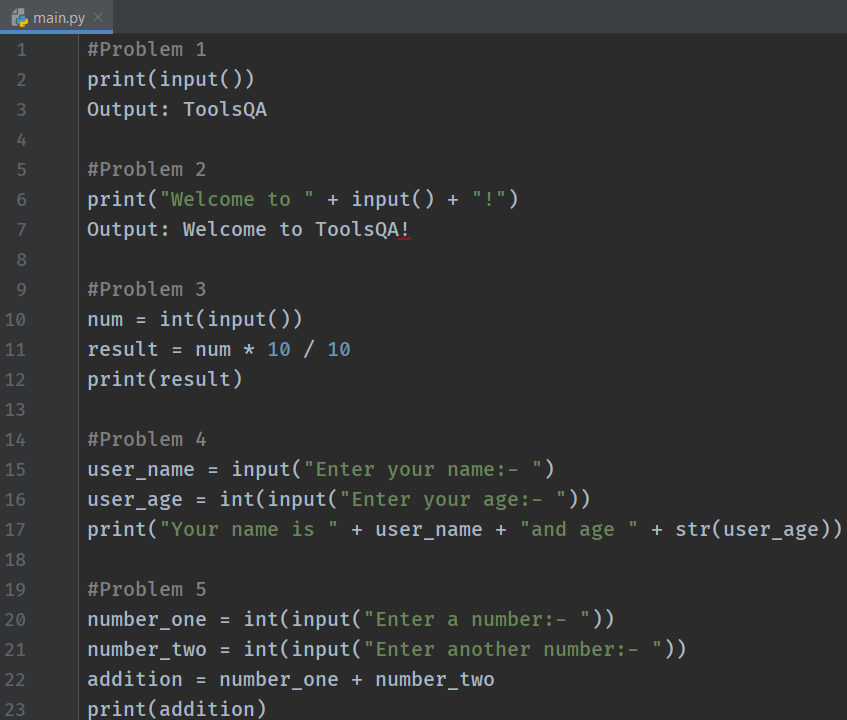### What Next?

To conclude, we have completed learning the Input/Output(I/O) functions in Python. Additionally, try to take different inputs using the input function and use the typecasting and practice. Remember - the more you practice, the more you will feel comfortable with Python coding.

In addition to the above, we have arithmetic operators + and * for integers. They have different meanings when you use it with string operands. Let's understand Python String Operator in detail in the next section.

Happy Coding :)_column_text][/vc_column][/vc_row]